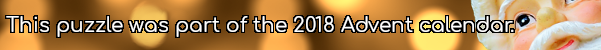mscroggs.co.uk
mscroggs.co.uksubscribe

# Puzzles

## 18 DecemberThere are 6 terms in the expansion of $$(x+y+z)^2$$:
$$(x+y+z)^2=x^2+y^2+z^2+2xy+2yz+2xz$$
Today's number is number of terms in the expansion of $$(x+y+z)^{16}$$.
Tags: algebra

## 10 DecemberThe equation $$x^2+1512x+414720=0$$ has two integer solutions.
Today's number is the number of (positive or negative) integers $$b$$ such that $$x^2+bx+414720=0$$ has two integer solutions.

Source: nrich
Find all real solutions to
$$(x^2-7x+11)^{(x^2-11x+30)}=1.$$

## Two tangents

Source: Reddit
Find a line which is tangent to the curve $$y=x^4-4x^3$$ at 2 points.

## A bit of Spanish

Each of the letters P, O, C, M, U and H represent a different digit from 0 to 9.
Which digit does each letter represent?

## Algebraic fractions

Given that
$$\frac{3x+y}{x-3y}=-1$$
what is the value of
$$\frac{x+3y}{3x-y}$$
?
Tags: algebra

## Four integers

$$a$$, $$b$$, $$c$$ and $$d$$ are four positive (and non-zero) integers.
$$abcd+abc+bcd+cda+dab+ab+bc+cd+da+ac+bd\\+a+b+c+d=2009$$
What is the value of $$a+b+c+d$$?

## Times roamin'

What is the product of this series?
$$(x-a)(x-b)(x-c)...(x-z)$$
Tags: algebra

## Archive

Show me a random puzzle
▼ show ▼